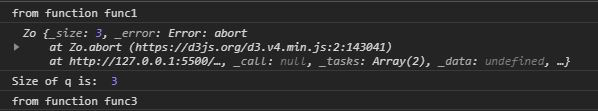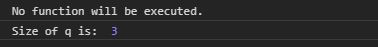# D3.js queue.abort() Function

• Last Updated : 04 Aug, 2020

The queue.abort() function in d3.js is used to abort every function of deferred tasks. If any deferred task does not return any abort than it will continue to execute further.

Syntax:

Hey geek! The constant emerging technologies in the world of web development always keeps the excitement for this subject through the roof. But before you tackle the big projects, we suggest you start by learning the basics. Kickstart your web development journey by learning JS concepts with our JavaScript Course. Now at it's lowest price ever!

```queue.abort();
```

Parameters: This function does not accept any parameter.

Returns: It returns the object.

Below given are a few examples of the above function.

Example 1: Certain function calls will be executed.

## HTML

 ` ``<``html` `lang``=``"en"``> ``<``head``> ``    ``<``meta` `charset``=``"UTF-8"``> ``    ``<``meta` `name``=``"viewport"``            ``path1tent="``width``=``device``-width, ``                       ``initial-scale``=``1``.0"> ``    ``<``title``>Document `` ``<``style``> `` ``<``body``> ``  ``<``script` `src` `= ``"https://d3js.org/d3.v4.min.js"``> ``  `` ``  ``<``script``>``    ``function func1(){``      ``console.log("from function func1")``    ``}``    ``function func2(){``      ``console.log("from function func2")``    ``}``    ``function func3(){``      ``console.log("from function func3")``    ``}``    ``function func4(){``      ``setTimeout(func3, 500);``    ``}``    ``let q=d3.queue(3)``    ``q.defer(func1)``    ``q.defer(func4)``    ``q.abort()``    ``// This will not be executed.``    ``q.defer(func2)``    ``console.log(q)``    ``console.log("Size of q is: ",q._size)``  `` `` ```

Output:Example 2: No function will be executed.

## HTML

 ` ``<``html` `lang``=``"en"``> ``<``head``> ``    ``<``meta` `charset``=``"UTF-8"``> ``    ``<``meta` `name``=``"viewport"``            ``path1tent="``width``=``device``-width, ``                       ``initial-scale``=``1``.0"> ``    ``<``title``>Document `` ``<``style``> `` ``<``body``> ``  ``<``script` `src` `= ``"https://d3js.org/d3.v4.min.js"``> ``  `` ``  ``<``script``>``    ``function func1(){``      ``console.log("from function func1")``    ``}``    ``function func2(){``      ``console.log("from function func2")``    ``}``    ``function func3(){``      ``console.log("from function func3")``    ``}``    ``function func4(){``      ``setTimeout(func3, 500);``    ``}``    ``let q=d3.queue(3)``    ``// Using abort function to force``    ``// every deferred function to return abort.``    ``q.abort()``    ``// These functions will not be executed.``    ``q.defer(func1)``    ``q.defer(func4)``    ``q.defer(func2)``    ``console.log("No function will be executed.")``    ``console.log("Size of q is: ",q._size)``  `` `` ```

Output:My Personal Notes arrow_drop_up